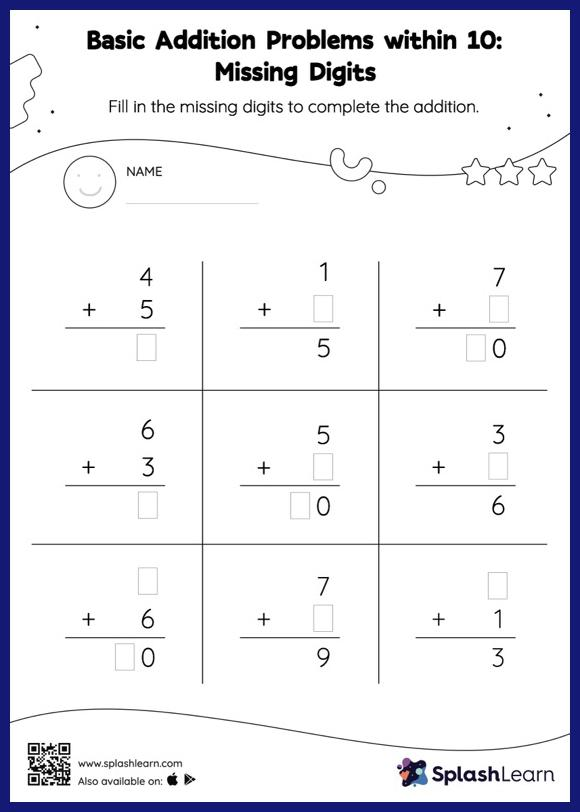# Basic Addition Problems within 10: Missing Digits Worksheet

Home > Basic Addition Problems within 10: Missing DigitsCan your young mathematician perform basic addition problems within 10? That's exactly what they do here. If an addend is missing, students use the count-on method or the relationship between addition and subtraction to determine the missing number. Also, they add the addends if the missing number is the sum of the addition sentence. This worksheet, basic addition problems within 10, offers a tonne of practice with this idea. This worksheet is about practicing the column method in which numbers are written one on top of another. In this method, students use their place value understanding to solve the problems.